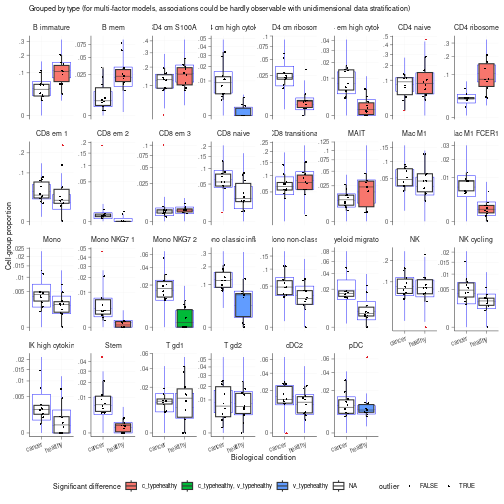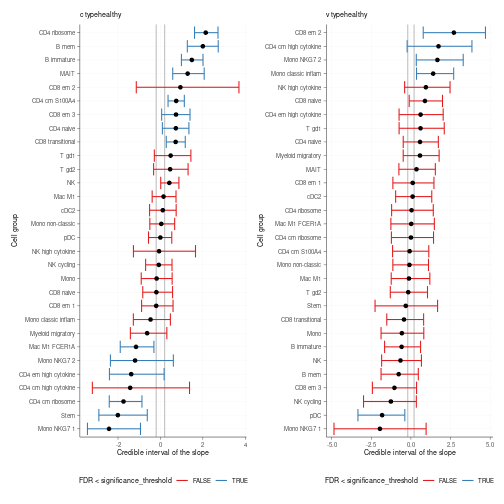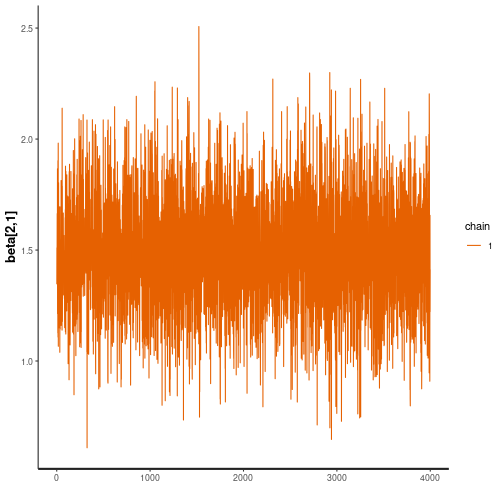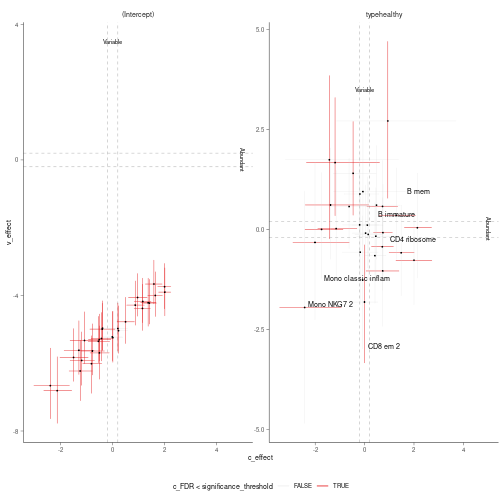#Sccomp is a generalised method for differential composition and variability analyses.

## Characteristics

• Modelling counts
• Modelling proportionality
• Modelling cell-type specific variability
• Cell-type information share for variability shrinkage
• Testing differential variability
• Probabilistic outlier identification
• Cross-dataset learning (hyperpriors).

# Installation

Bioconductor

``````if (!requireNamespace("BiocManager")) install.packages("BiocManager")
BiocManager::install("sccomp")
``````

Github

``````devtools::install_github("stemangiola/sccomp")
``````

# Analysis

`sccomp` can model changes in composition and variability. By default, the formula for variability is either `~1`, which assumes that the cell-group variability is independent of any covariate or `~ factor_of_interest`, which assumes that the model is dependent on the factor of interest only. The variability model must be a subset of the model for composition.

## Binary factor

### From Seurat, SingleCellExperiment, metadata objects

``````single_cell_object |>
sccomp_glm(
formula_composition = ~ type,
.sample =  sample,
.cell_group = cell_group,
bimodal_mean_variability_association = TRUE,
cores = 1
)
``````

### From counts

``````counts_obj |>
sccomp_glm(
formula_composition = ~ type,
.sample = sample,
.cell_group = cell_group,
.count = count,
bimodal_mean_variability_association = TRUE,
cores = 1
)
``````
``````## # A tibble: 72 × 18
##    cell_group parameter  factor c_lower c_effect c_upper   c_pH0   c_FDR c_n_eff
##    <chr>      <chr>      <chr>    <dbl>    <dbl>   <dbl>   <dbl>   <dbl>   <dbl>
##  1 B1         (Intercep… <NA>     0.873    1.06   1.24   0       0         4721.
##  2 B1         typecancer type    -1.22    -0.869 -0.534  2.50e-4 5.00e-5   2317.
##  3 B2         (Intercep… <NA>     0.415    0.697  0.950  1.00e-3 6.25e-5   4452.
##  4 B2         typecancer type    -1.20    -0.773 -0.353  4.75e-3 7.22e-4   3174.
##  5 B3         (Intercep… <NA>    -0.628   -0.381 -0.138  6.97e-2 3.74e-3   4050.
##  6 B3         typecancer type    -0.647   -0.232  0.135  4.29e-1 1.15e-1   2843.
##  7 BM         (Intercep… <NA>    -1.29    -1.01  -0.745  0       0         4299.
##  8 BM         typecancer type    -0.751   -0.345  0.0452 2.27e-1 4.97e-2   3492.
##  9 CD4 1      (Intercep… <NA>     0.121    0.322  0.519  1.08e-1 1.14e-2   3088.
## 10 CD4 1      typecancer type    -0.110    0.160  0.440  6.10e-1 2.22e-1   2962.
## # ℹ 62 more rows
## # ℹ 9 more variables: c_R_k_hat <dbl>, v_lower <dbl>, v_effect <dbl>,
## #   v_upper <dbl>, v_pH0 <dbl>, v_FDR <dbl>, v_n_eff <dbl>, v_R_k_hat <dbl>,
## #   count_data <list>
``````

Of the output table, the estimate columns start with the prefix `c_` indicate `composition`, or with `v_` indicate `variability` (when formula_variability is set).

## Contrasts

``````seurat_obj |>
sccomp_glm(
formula_composition = ~ 0 + type,
contrasts =  c("typecancer - typehealthy", "typehealthy - typecancer"),
.sample = sample,
.cell_group = cell_group,
bimodal_mean_variability_association = TRUE,
cores = 1
)
``````
``````## # A tibble: 60 × 18
##    cell_group  parameter factor c_lower c_effect c_upper   c_pH0   c_FDR c_n_eff
##    <chr>       <chr>     <chr>    <dbl>    <dbl>   <dbl>   <dbl>   <dbl>   <dbl>
##  1 B immature  typecanc… type    -2.01    -1.57   -1.13  0       0            NA
##  2 B immature  typeheal… type     1.13     1.57    2.01  0       0            NA
##  3 B mem       typecanc… type    -2.53    -1.93   -1.29  0       0            NA
##  4 B mem       typeheal… type     1.29     1.93    2.53  0       0            NA
##  5 CD4 cm S10… typecanc… type    -1.25    -0.875  -0.524 0       0            NA
##  6 CD4 cm S10… typeheal… type     0.524    0.875   1.25  0       0            NA
##  7 CD4 cm hig… typecanc… type     0.946    1.87    2.98  5.00e-4 9.37e-5      NA
##  8 CD4 cm hig… typeheal… type    -2.98    -1.87   -0.946 5.00e-4 9.37e-5      NA
##  9 CD4 cm rib… typecanc… type     0.590    1.24    1.97  2.50e-4 3.57e-5      NA
## 10 CD4 cm rib… typeheal… type    -1.97    -1.24   -0.590 2.50e-4 3.57e-5      NA
## # ℹ 50 more rows
## # ℹ 9 more variables: c_R_k_hat <dbl>, v_lower <dbl>, v_effect <dbl>,
## #   v_upper <dbl>, v_pH0 <dbl>, v_FDR <dbl>, v_n_eff <dbl>, v_R_k_hat <dbl>,
## #   count_data <list>
``````

## Categorical factor (e.g. Bayesian ANOVA)

This is achieved through model comparison with `loo`. In the following example, the model with association with factors better fits the data compared to the baseline model with no factor association. For comparisons `check_outliers` must be set to FALSE as the leave-one-out must work with the same amount of data, while outlier elimination does not guarantee it.

If `elpd_diff` is away from zero of > 5 `se_diff` difference of 5, we are confident that a model is better than the other reference. In this case, -79.9 / 11.5 = -6.9, therefore we can conclude that model one, the one with factor association, is better than model two.

``````library(loo)

# Fit first model
model_with_factor_association =
seurat_obj |>
sccomp_glm(
formula_composition = ~ type,
.sample =  sample,
.cell_group = cell_group,
check_outliers = FALSE,
bimodal_mean_variability_association = TRUE,
cores = 1,
enable_loo = TRUE
)

# Fit second model
model_without_association =
seurat_obj |>
sccomp_glm(
formula_composition = ~ 1,
.sample =  sample,
.cell_group = cell_group,
check_outliers = FALSE,
bimodal_mean_variability_association = TRUE,
cores = 1 ,
enable_loo = TRUE
)

# Compare models
loo_compare(
model_with_factor_association |> attr("fit") |> loo(),
model_without_association |> attr("fit") |> loo()
)
``````

## Differential variability, binary factor

We can model the cell-group variability also dependent on the type, and so test differences in variability

``````res =
seurat_obj |>
sccomp_glm(
formula_composition = ~ type,
formula_variability = ~ type,
.sample = sample,
.cell_group = cell_group,
bimodal_mean_variability_association = TRUE,
cores = 1
)

res
``````
``````## # A tibble: 60 × 18
##    cell_group  parameter factor c_lower c_effect c_upper   c_pH0   c_FDR c_n_eff
##    <chr>       <chr>     <chr>    <dbl>    <dbl>   <dbl>   <dbl>   <dbl>   <dbl>
##  1 B immature  (Interce… <NA>     0.607    0.963   1.33  0       0         6757.
##  2 B immature  typeheal… type     0.990    1.48    2.01  0       0         4360.
##  3 B mem       (Interce… <NA>    -1.64    -1.08   -0.495 0.00175 1.81e-4   5180.
##  4 B mem       typeheal… type     1.27     2.00    2.73  0       0         4083.
##  5 CD4 cm S10… (Interce… <NA>     1.77     2.02    2.25  0       0         5626.
##  6 CD4 cm S10… typeheal… type     0.359    0.745   1.13  0.00250 6.00e-4   3493.
##  7 CD4 cm hig… (Interce… <NA>    -0.838   -0.385   0.110 0.22    3.12e-2   3845.
##  8 CD4 cm hig… typeheal… type    -3.21    -1.43    1.37  0.182   4.86e-2   2957.
##  9 CD4 cm rib… (Interce… <NA>     0.169    0.500   0.845 0.0370  2.44e-3   4944.
## 10 CD4 cm rib… typeheal… type    -2.41    -1.74   -0.871 0.00300 1.00e-3   2608.
## # ℹ 50 more rows
## # ℹ 9 more variables: c_R_k_hat <dbl>, v_lower <dbl>, v_effect <dbl>,
## #   v_upper <dbl>, v_pH0 <dbl>, v_FDR <dbl>, v_n_eff <dbl>, v_R_k_hat <dbl>,
## #   count_data <list>
``````

# Suggested settings

## For single-cell RNA sequencing

We recommend setting `bimodal_mean_variability_association = TRUE`. The bimodality of the mean-variability association can be confirmed from the plots\$credible_intervals_2D (see below).

## For CyTOF and microbiome data

We recommend setting `bimodal_mean_variability_association = FALSE` (Default).

# Visualisation

## Summary plots

``````plots = plot_summary(res)
``````
``````## Joining with `by = join_by(cell_group, sample)`
## Joining with `by = join_by(cell_group, type)`
``````
``````## Warning: Expected 2 pieces. Additional pieces discarded in 4 rows [6, 7, 13,
## 14].
``````

A plot of group proportion, faceted by groups. The blue boxplots represent the posterior predictive check. If the model is likely to be descriptively adequate to the data, the blue box plot should roughly overlay with the black box plot, which represents the observed data. The outliers are coloured in red. A box plot will be returned for every (discrete) covariate present in `formula_composition`. The colour coding represents the significant associations for composition and/or variability.

``````plots\$boxplot
``````
``````## []
``````A plot of estimates of differential composition (c_) on the x-axis and differential variability (v_) on the y-axis. The error bars represent 95% credible intervals. The dashed lines represent the minimal effect that the hypothesis test is based on. An effect is labelled as significant if bigger than the minimal effect according to the 95% credible interval. Facets represent the covariates in the model.

``````plots\$credible_intervals_1D
``````## Visualisation of the MCMC chains from the posterior distribution

It is possible to directly evaluate the posterior distribution. In this example, we plot the Monte Carlo chain for the slope parameter of the first cell type. We can see that it has converged and is negative with probability 1.

``````res %>% attr("fit") %>% rstan::traceplot("beta[2,1]")
``````Plot 1D significance plot

``````plots = plot_summary(res)
``````
``````## Joining with `by = join_by(cell_group, sample)`
## Joining with `by = join_by(cell_group, type)`
``````
``````## Warning: Expected 2 pieces. Additional pieces discarded in 4 rows [6, 7, 13,
## 14].
``````
``````plots\$credible_intervals_1D
``````Plot 2D significance plot. Data points are cell groups. Error bars are the 95% credible interval. The dashed lines represent the default threshold fold change for which the probabilities (c_pH0, v_pH0) are calculated. pH0 of 0 represent the rejection of the null hypothesis that no effect is observed.

This plot is provided only if differential variability has been tested. The differential variability estimates are reliable only if the linear association between mean and variability for `(intercept)` (left-hand side facet) is satisfied. A scatterplot (besides the Intercept) is provided for each category of interest. The for each category of interest, the composition and variability effects should be generally uncorrelated.

``````plots\$credible_intervals_2D
````````````sessionInfo()
``````
``````## R version 4.3.0 RC (2023-04-13 r84269)
## Platform: x86_64-pc-linux-gnu (64-bit)
## Running under: Ubuntu 22.04.2 LTS
##
## Matrix products: default
## BLAS:   /home/biocbuild/bbs-3.17-bioc/R/lib/libRblas.so
## LAPACK: /usr/lib/x86_64-linux-gnu/lapack/liblapack.so.3.10.0
##
## locale:
##   LC_CTYPE=en_US.UTF-8       LC_NUMERIC=C
##   LC_TIME=en_GB              LC_COLLATE=C
##   LC_MONETARY=en_US.UTF-8    LC_MESSAGES=en_US.UTF-8
##   LC_PAPER=en_US.UTF-8       LC_NAME=C
##  LC_MEASUREMENT=en_US.UTF-8 LC_IDENTIFICATION=C
##
## time zone: America/New_York
## tzcode source: system (glibc)
##
## attached base packages:
##  stats     graphics  grDevices utils     datasets  methods   base
##
## other attached packages:
##  forcats_1.0.0        ggplot2_3.4.2        sccomp_1.4.0
##  dplyr_1.1.2
##
## loaded via a namespace (and not attached):
##   tidyselect_1.2.0            farver_2.1.1
##   loo_2.6.0                   bitops_1.0-7
##   SingleCellExperiment_1.22.0 RCurl_1.98-1.12
##   digest_0.6.31               lifecycle_1.0.3
##   SeuratObject_4.1.3          processx_3.8.1
##  magrittr_2.0.3              compiler_4.3.0
##  rlang_1.1.0                 tools_4.3.0
##  utf8_1.2.3                  knitr_1.42
##  labeling_0.4.2              prettyunits_1.1.1
##  sp_1.6-0                    pkgbuild_1.4.0
##  DelayedArray_0.26.0         RColorBrewer_1.1-3
##  withr_2.5.0                 purrr_1.0.1
##  BiocGenerics_0.46.0         grid_4.3.0
##  stats4_4.3.0                fansi_1.0.4
##  colorspace_2.1-0            future_1.32.0
##  inline_0.3.19               progressr_0.13.0
##  globals_0.16.2              scales_1.2.1
##  SummarizedExperiment_1.30.0 cli_3.6.1
##  crayon_1.5.2                generics_0.1.3
##  RcppParallel_5.1.7          future.apply_1.10.0
##  tzdb_0.3.0                  stringr_1.5.0
##  zlibbioc_1.46.0             parallel_4.3.0
##  XVector_0.40.0              matrixStats_0.63.0
##  vctrs_0.6.2                 boot_1.3-28.1
##  Matrix_1.5-4                callr_3.7.3
##  IRanges_2.34.0              hms_1.1.3
##  patchwork_1.1.2             S4Vectors_0.38.0
##  ggrepel_0.9.3               listenv_0.9.0
##  glue_1.6.2                  parallelly_1.35.0
##  codetools_0.2-19            ps_1.7.5
##  stringi_1.7.12              gtable_0.3.3
##  GenomeInfoDb_1.36.0         GenomicRanges_1.52.0
##  munsell_0.5.0               tibble_3.2.1
##  pillar_1.9.0                GenomeInfoDbData_1.2.10
##  R6_2.5.1                    evaluate_0.20
##  lattice_0.21-8              Biobase_2.60.0